## Figures index

#### Modeling and Optimization of Dynamical Systems by Unconventional Spreadsheet Functions

American Journal of Modeling and Optimization. 2016, 4(1), 1-12 doi:10.12691/ajmo-4-1-1• Figure 1. Flow chart for unconventional first class spreadsheet function design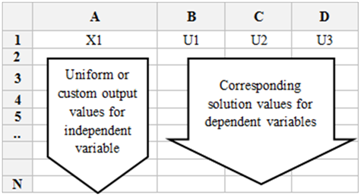• Figure 2. Solution layout in Excel for differential systems solvers IVSOLVE() and BVSOLVE()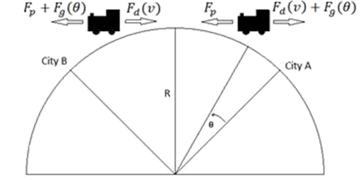• Figure 3. Schematic for the forces acting on the travelling train of problem 3.1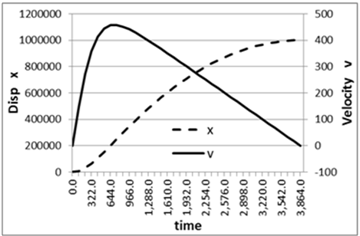• Figure 4. Simulation of train motion using optimal parameters of Table 7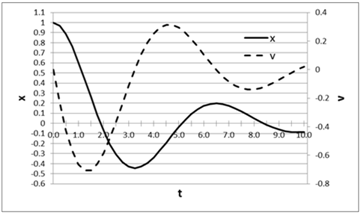• Figure 5. Plot of system response computed in Table 9 using the parameters values given in Table 8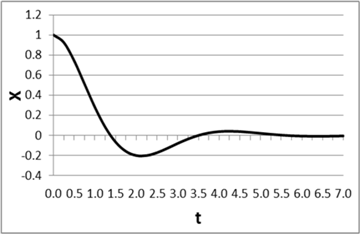• Figure 6. Modified system response using optimal parameters of Table 11• Figure 7. Modified system response using parameters of Table 12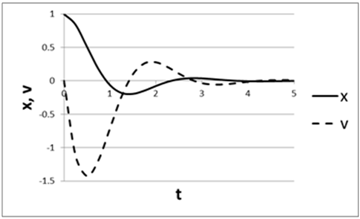• Figure 8. Modified system response using parameters of Table 14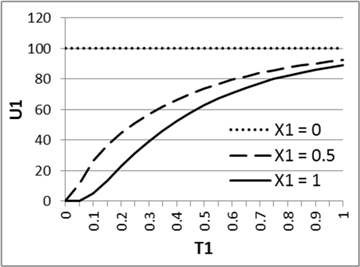• Figure 9A.Transient plot of the system response computed in Table 16 at different spatial points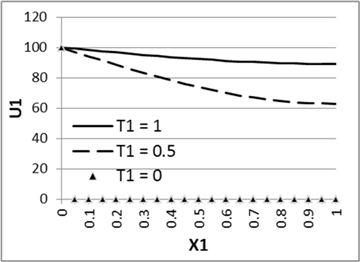• Figure 9B. Snapshot plot shows the temperature spatial profile at different times• Figure 10. Plot for results of Table 20 for boundary value problem (A.4)• Figure 11.Snapshot solution layout in Excel for partial differential equation solver PDSOLVE(). The display of 1st and 2nd derivative variables is optional